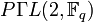# Projective semilinear group of degree two

(diff) ← Older revision | Latest revision (diff) | Newer revision → (diff)

## Definition

Suppose$K$ is a field. The projective semilinear group of degree two over$K$ is defined as the projective semilinear group of degree two over$K$. It is denoted$P\Gamma L(2,K)$.

It can be described as an external semidirect product of the projective general linear group of degree two over$K$ by the Galois group of$K$ over its prime subfield$k$, where the latter acts on the former by applying the Galois automorphism to all the matrix entries in any representing matrix:$P\Gamma L(2,K) = PGL(2,K) \rtimes \operatorname{Gal}(K/k)$

In the particular case that$K$ is a prime field (i.e., either a field of prime size or the field of rational numbers),$P\Gamma L(2,K)$ can be identified with$PGL(2,K)$.

For a prime power$q$, we denote by$P\Gamma L(2,q)$ the group$P\Gamma L(2,\mathbb{F}_q)$, where$\mathbb{F}_q$ is the (unique up to isomorphism) field of size$q$.

## Arithmetic functions

### Over finite field

We consider the case where$K$ is the (unique up to isomorphism) field of size$q$, with$q = p^r$, so$p$ is the field characteristic and$r$ is the order of the Galois group$\operatorname{Gal}(K/k)$.

Function Value Similar groups Explanation
order$r(q^3 - q) = rq(q+1)(q-1)$ -- order of semidirect product is product of orders: the order of$PGL(2,q)$ is$q^3 - q$ and the order of$\operatorname{Gal}(K/k)$ is$r$.

## Particular cases$q$ (field size)$p$ (underlying prime, field characteristic)$r$ (size of Galois group)$P\Gamma L(2,q)$ Order of$P\Gamma L(2,q)$ (=$r(q^3 - q)$)
2 2 1 symmetric group:S3 6
3 3 1 symmetric group:S4 24
4 2 2 symmetric group:S5 120
5 5 1 symmetric group:S5 120
7 7 1 projective general linear group:PGL(2,7) 336
8 2 3 Ree group:Ree(3) 1512
9 3 2 automorphism group of alternating group:A6 1440
11 11 1 projective general linear group:PGL(2,11) 1320